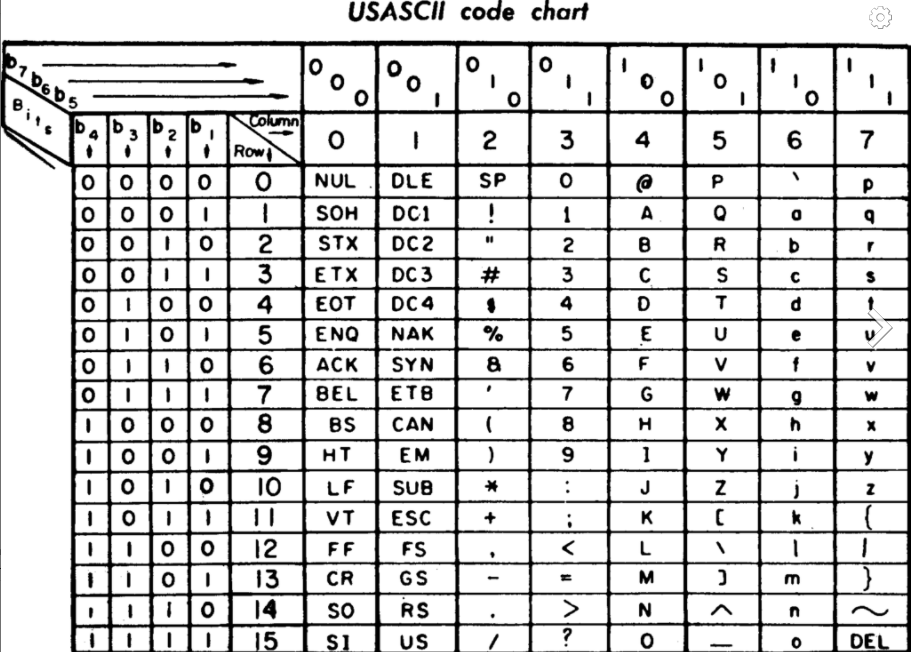## Asterisk. /ˈastərɪsk/The asterisk, from latin asteriscus, from ancient greek ἀστερίσκος, asteriskos, “little star” is a typographical symbol (*) used in text as a pointer to an annotation or footnote.

In economics, by convention, adding an asterisk to a variable, makes it optimal, makes it the one and only solution to a problem.

In the 1960’s, the American Standard Code for Information Interchange (ASCII) was created as a new character encoding standard for electronic communication. Most modern character-encoding schemes are based on ASCII and nowadays our day-to-day keyboard Alt short codes are directly coming from the 1960’s ASCII standard.

The code used for the asterisk as determined by ASCII was set to the number 42.

In 1979, Douglas Adams published a sci-fi book called The Hitchhiker’s Guide to the Galaxy, where the number 42 is presented as “the answer to the ultimate question of life, the universe, and everything.”

More recently, one of the most famous mathematical puzzle found its solution in relation to the number 42.

The original problem, set in 1954 by University of Cambridge researchers, looked for solutions of the Diophantine equation x3 + y3 + z3 = k, with k being all the numbers from one to 100.

Beyond the easily found solutions, the problem soon became a significant puzzle for some of the most sophisticated mathematicians as some answers could not possibly be calculated (given the power of calculations it required). But years after years, thanks to modern computers and improved calculations techniques, each value of k was eventually found (or demonstrated unsolvable) — except the last two, the most difficult of all: 33 and 42.

Until in 2019, when British mathematician Andrew Booker, professor of pure mathematics at the University of Bristol finally found an answer for 33.

However 42 remained the unsolved puzzle. Until professor Booker, in late 2019, reached out to Charity Engine, a “planetary computer” that offers crowd-sourced, low carbon platform of unused computing power. Millions hours of calculation later, the answer to the Diophine equation “x3 + y3 + z3 = 42” was found:

(-80538738812075974)3 + 804357581458175153+ 126021232973356313 = 42

This list of unrelated events was enough to establish 42 as a particular number to TOBAM. One that has now some magic.